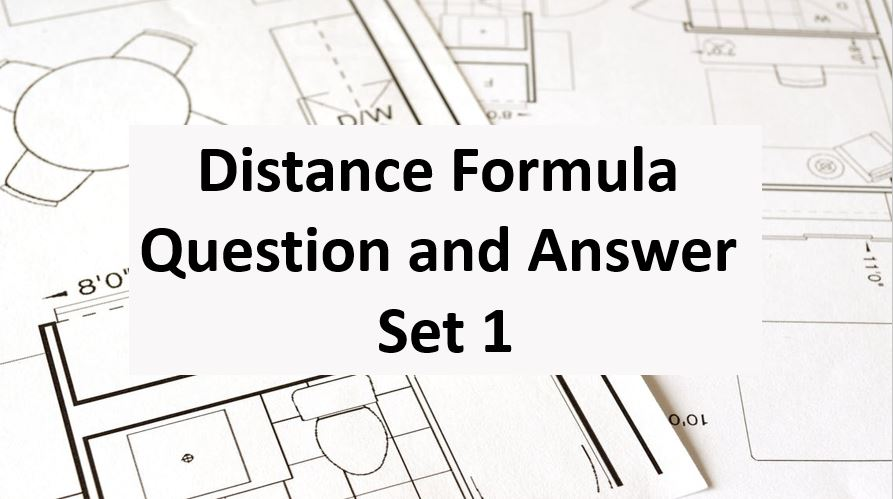Friday, October 22, 2021
Home > Question and Answer > Distance Formula Question And Answer Set 1

# Distance Formula Question And Answer Set 1# Distance Formula Question and Answer Set 1

Hi students, welcome to Amans Maths Blogs (AMB). On this post, you will get the Distance Formula Question and Answer Set 1 is the collection of some important questions. Practice these questions for SSC CGL CHSL CAT NTSE exams etc. It will help you to practice the questions on the topics of maths as distance formula based questions of coordinate geometry.

Distance Formula Question and Answer Set 1: Ques No 1

If (x, y), (1, 1) and (5, 1) are the coordinates of a right triangle with the hypotenuse defined by the points (1, 1) and (5, 1), then the relationship between x and y is

Options:

A. (x + 3)2 + (y – 1)2 = 9

B. (x – 2)2 + (y – 1)2 = 16

C. (x – 3)2 + (y + 1)2 = 25

D. (x – 3)2 + (y – 1)2 = 4

Distance Formula Question and Answer Set 1: Ques No 2

The relationship between x and y so that (x, y) is equidistant from the two points (-3, 4) and (0, -3) is

Options:

A. 6x + 14 y + 16 = 0

B. 6x – 14 y + 16 = 0

C. 6x – 14 y – 16 = 0

D. 6x + 14 y – 16 = 0

Distance Formula Question and Answer Set 1: Ques No 3

The distance between (k,0) and (0, 2k) is 10 units, where k > 0. The value of k is

Options:

A. 2Root(5)

B. 5Root(3)

C. 4Root(3)

D. 3Root(5)

Distance Formula Question and Answer Set 1: Ques No 4

The relationship between x and y so that the distance between the points (x, y) and (-2, 4) is equal to 5

Options:

A. x2 – y2 + 4x – 8y = 15

B. x2 + y2 + 4x + 8y = 7

C. x2 + y2 + 4x – 8y = 5

D. x2 – y2 – 4x + 8y = 8

Distance Formula Question and Answer Set 1: Ques No 5

The coordinates of the vertices of a triangle are (0, 1), (2, 3) and (2, -1). The triangle is a

Options:

A. Isosceles Triangle

B. Right Triangle

C. Equilateral Triangle

D. Right Isosceles Triangle

Distance Formula Question and Answer Set 1: Ques No 6

The distance between point (-1, -3) and the midpoint of the line segment joining (2, 4) and (4, 6) is

Options:

A. 4Root(5)

B. 3Root(2)

C. 2Root(3)

D. 4Root(2)

Distance Formula Question and Answer Set 1: Ques No 7

The  distance between the points (2, 3) and (0, 6) is

Options:

A. Root(10)

B. Root(13)

C. Root(12)

D. Root(11)